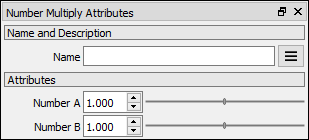# Number Multiply node

Left Toolbar >Math Nodes >Number Multiply

The Number Multiply node allows you to execute a multiplication operation on multiple Number values.

## Procedure

### To execute a multiplication operation on multiple Node Values in the node graph:

1. On the left toolbar choose Math Nodes> Number Multiply2. Drag the Number Multiply icon into the Node Graph.3. Connect the Output: result.

## Inputs

number A NUMBER type of value. This input grows automatically when a value is connected.

## Outputs

result The number value.

## Attributes Panelnumber_a The first term in the multiplication operation. This attribute is exposed as input by default.

number_b The second term in the multiplication operation. This attribute is exposed as input by default.

Math Nodes

Main Page